Chapter 2 National Income - Part 5 Expenditure Method

Economics Class 12
Macroeconomics

As per this Method

We calculate total final expenditure of different sectors of economy

We reduce Depreciation and Net Indirect taxes to arrive at NDP at FC

Steps followed

Step 1

Identify Different Sectors of economy and calculate their Final Expenditure

Step 2

Calculate Total Expenditure of all Sectors to arrive at GDP at MP

Step 3

We reduce Depreciation and Net Indirect taxes to arrive at NDP at FC (Domestic Income)

Step 4

We add NFIA to get National Income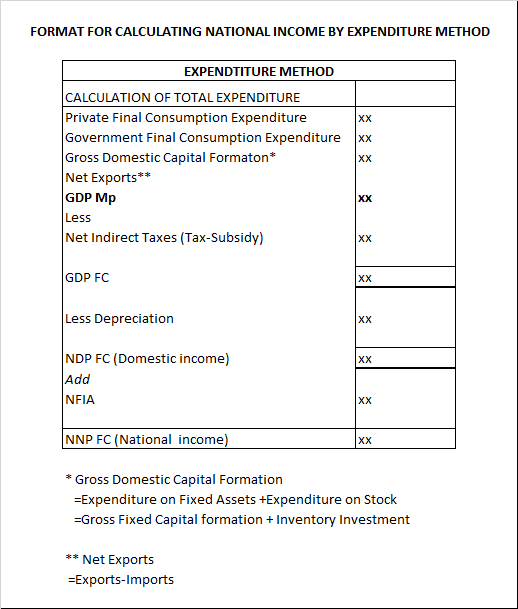Example 27

Calculate GDP at MP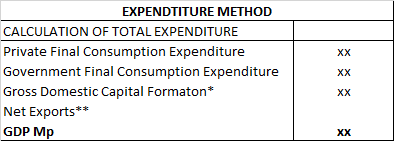Step 1

We calculate Gross Domestic Capital Formation

* Gross Domestic Capital Formation

=Expenditure on Fixed Assets +Expenditure on Stock

=Gross Fixed Capital formation + Inventory Investment

300 + 400 = 700

Step 2

We calculate Net Exports

Net Exports

=Exports -Imports

600-500 =100

Step 3

We calculate GDP Mp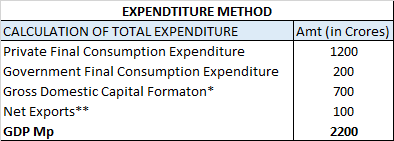Question 29

Calculate National Income.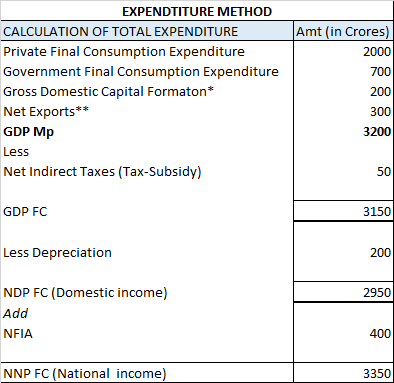#### Note

Consumption of Fixed Capital is the other name of Depreciation

Depreciation =Consumption of Fixed Capital = 700

Question 30

Calculate National Income from the following data: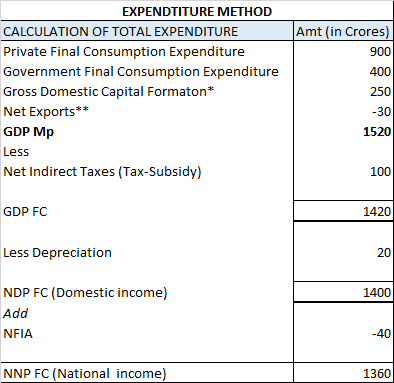#### Note

Here, we are given Net Imports instead of Net Exports

Net Imports =30

So Net Export = - 30

Learn in your speed, with individual attention - Teachoo Maths 1-on-1 Class